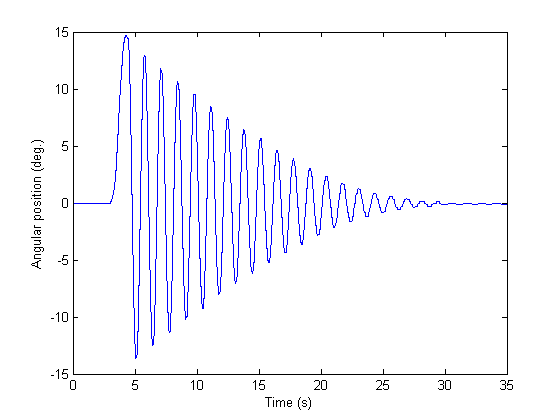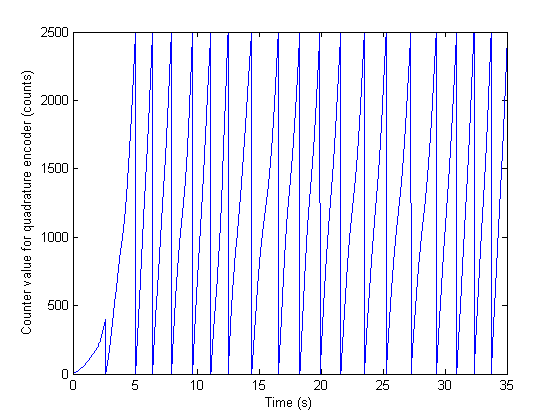## Measure Angular Position with an Incremental Rotary Encoder

This example shows how to acquire angular position data using an incremental rotary encoder and a multifunction data acquisition (DAQ) device with the Data Acquisition Toolbox quadrature encoder measurement functionality.

An incremental rotary encoder is typically mounted on the shaft of a mechanical system, such as a wind turbine or a robotic arm, to provide motion or position information. The encoder outputs two quadrature signals, which provide information on the relative change in position and the direction of rotation. The counter subsystem of the DAQ device uses the signals output by the encoder to calculate the change in position, and keep track of the most recent position value. In MATLAB, a counter input channel is used to read the position values.

This example uses an optical shaft encoder (US Digital H6-2500-IE-S) and a multifunction DAQ device (NI USB-6255) with counter channels which have quadrature encoder capability.

### Create a data acquisition session

Create a session for `ni` and add a counter input channel for `Position` measurement type.

```s = daq.createSession('ni'); ch1 = addCounterInputChannel(s, 'Dev1', 0, 'Position') ```
```ch1 = Data acquisition counter input position channel 'ctr0' on device 'Dev1': EncoderType: X1 ZResetEnable: 0 ZResetValue: 0 ZResetCondition: BothHigh TerminalA: 'PFI8' TerminalB: 'PFI10' TerminalZ: 'PFI9' Name: '' ID: 'ctr0' Device: [1x1 daq.ni.DeviceInfo] MeasurementType: 'Position' ```

### Configure hardware

A rotary quadrature encoder outputs two quadrature signals, A and B, which provide information on the relative change in position and the direction of rotation. Optionally, some models also output an index or reference signal, Z, which is active once per revolution. You can use this to reset the counter position to a known reference value.

Connect A, B, and Z signal outputs to the proper input terminals specified by the DAQ device datasheet (PFI8, PFI10, and PFI9 for NI USB-6255).

```ch1.TerminalA ch1.TerminalB ch1.TerminalZ ```
```ans = PFI8 ans = PFI10 ans = PFI9 ```

Configure quadrature cycle encoding type (X1, X2, or X4). This corresponds to the number of counts (counter value increments or decrements) for each quadrature cycle (1, 2, or 4.)

```ch1.EncoderType = 'X1'; ```

### Read encoder position on demand

The DAQ device counter hardware keeps track of the relative position changes signaled by the encoder. Use `inputSingleScan` to read an updated position from the counter input channel.

```encoderPosition = inputSingleScan(s) ```
```encoderPosition = 0 ```

This example uses an optical encoder model with a resolution of 2500 quadrature cycles per shaft revolution, as specified in the encoder datasheet.

Convert counter values to angular position (in degrees) using the encoder resolution and the encoding type ('X1' in this case).

```encoderCPR = 2500; encoderPositionDeg = encoderPosition * 360/encoderCPR ```
```encoderPositionDeg = 0 ```

### Acquire hardware-timed encoder position data

For applications where high time-resolution is required the data acquisition must be hardware-timed (clocked). As proof of concept, this example characterizes the motion of a swinging pendulum by measuring its angular position vs. time.

To acquire hardware-timed data from a counter input channel, NI devices require the use of an external clock or the use of a clock from another subsystem.

```addAnalogInputChannel(s, 'Dev1', 0, 'Voltage'); ```

Configure acquisition rate (samples/s) and acquisition duration in seconds.

```s.Rate = 10000; s.DurationInSeconds = 35; ```

Acquire data in the foreground.

```[positionData, timestamps] = startForeground(s); ```

By default, counter position readings are unsigned integer values. The counter channels of the DAQ device used in this example are 32-bit, so any counter value read will be in the range 0 to 2^32-1. Depending on the application, you may want to obtain signed position values (positive or negative) as decrementing the counter value past zero is a discontinuous wraparound to 2^32-1.

For 32-bit counter channels, use 2^31 as the threshold counter value for conversion to signed position values. The result is valid if the actual position value is in the range -2^31+1 to 2^31.

```counterNBits = 32; signedThreshold = 2^(counterNBits-1); signedData = positionData(:,1); signedData(signedData > signedThreshold) = signedData(signedData > signedThreshold) - 2^counterNBits; ```

Calculate encoder position data in degrees.

```positionDataDeg = signedData * 360/encoderCPR; ```

Plot the signed angular position data acquired for the oscillatory motion of a pendulum.

```figure; plot(timestamps, positionDataDeg); xlabel('Time (s)'); ylabel('Angular position (deg.)'); ```### Use the Z signal to reference the relative position to a known absolute position

The A and B quadrature signals output by incremental rotary encoders provide only relative position information (direction of motion and changes in position). The optional reference signal Z is a single pulse output once per encoder shaft revolution at a predefined absolute location. Referencing the relative position value to the known absolute position reference allows an incremental rotary encoder to function as a pseudo-absolute position encoder. This is useful in accurate positioning applications (such as industrial automation, robotics, solar tracking, radar antenna or telescope positioning).

For incremental rotary encoders that provide a Z index signal output, the counter position value can be configured to be reset automatically to the known reference value.

Set the `ZResetEnable` and `ZResetCondition` properties.

```ch1.ZResetEnable = true; ```

Configure the `ZResetCondition`, which is based on the A and B phase signals. Possible values are:

• 'BothLow'

• 'BothHigh'

• 'AHigh'

• 'BHigh'

```ch1.ZResetCondition = 'BothLow'; ```

Specify the absolute reference position value `ZResetValue` to which the counter value will be reset.

```ch1.ZResetValue = 0; ```

Acquire and plot a set of hardware-timed counter position data to show how you can use the encoder Z index signal to automatically reset the counter value to a known reference value.

```[positionData2, timestamps2] = startForeground(s); figure; plot(timestamps2, positionData2(:,1)); xlabel('Time (s)'); ylabel('Counter value for quadrature encoder (counts)'); ```The acquired position data corresponds to a rotary encoder shaft that is rotating continuously. Notice how before the first time the counter value is reset the position value is not referenced to an absolute position, whereas the other counter reset events occur when the counter value is 2500 (the encoder CPR value).

##### SupportGet trial now